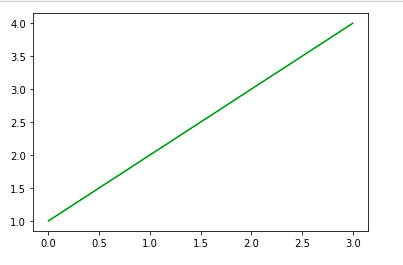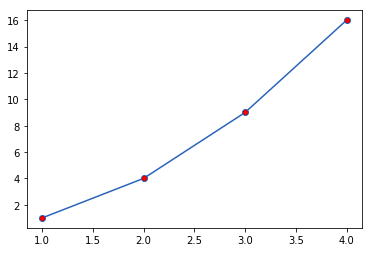Skip to content
Related Articles
Matplotlib.pyplot.colors() in Python
• Last Updated : 03 May, 2020

In Python we can plot graphs for visualization using Matplotlib library. For integrating plots into applications, Matplotlib provides an API. Matplotlib has a module named pyplot which provides a MATLAB-like interface.

## Matplotlib.pyplot.colors()

This function is used to specify the color. It is do-nothing function.

AliasColor
‘b’Blue
‘r’Red
‘g’Green
‘c’Cyan
‘m’Magenta
‘y’Yellow
‘k’Black
‘w’White

We can use this function for various data visualizations and obtain insights from them.

Examples 1:

 `import` `matplotlib.pyplot as plt`` ` ` ` `# Define the Color``color ``=` `'green'``plt.plot([``1``, ``2``, ``3``, ``4``], color ``=` `color)`` ` `plt.show()`

Output:Example 2:

 `import` `matplotlib.pyplot as plt`` ` ` ` `x ``=` `[``1``, ``2``, ``3``, ``4``]``y``=` `[``1``, ``4``, ``9``, ``16``]`` ` `plt.plot(x, y, marker ``=` `'o'``, markerfacecolor ``=` `'r'``)`` ` `plt.show()`

Output:Here, marker=’o’ represents circle while markerfacecolor is used for specifying color of the point marker.

Note: This function has been deprecated since version 2.1.

Attention geek! Strengthen your foundations with the Python Programming Foundation Course and learn the basics.

To begin with, your interview preparations Enhance your Data Structures concepts with the Python DS Course. And to begin with your Machine Learning Journey, join the Machine Learning – Basic Level Course

My Personal Notes arrow_drop_up